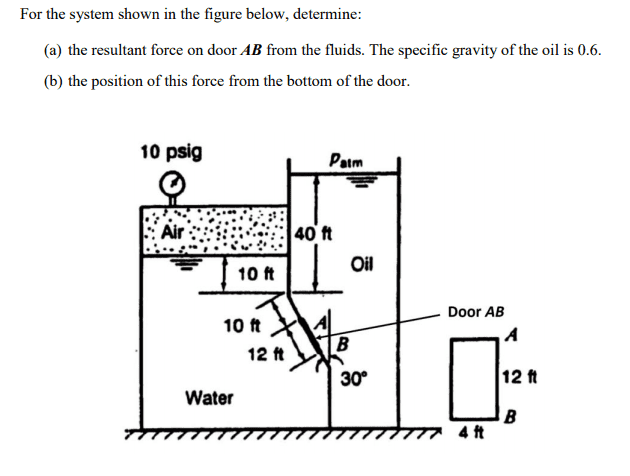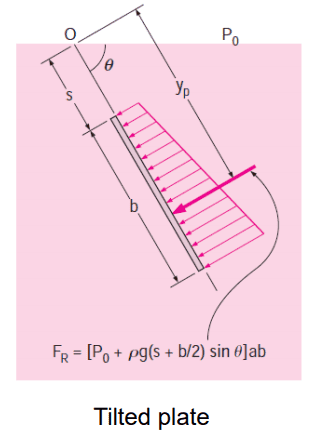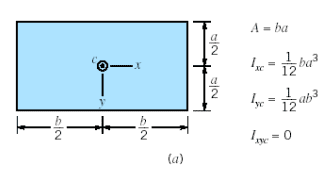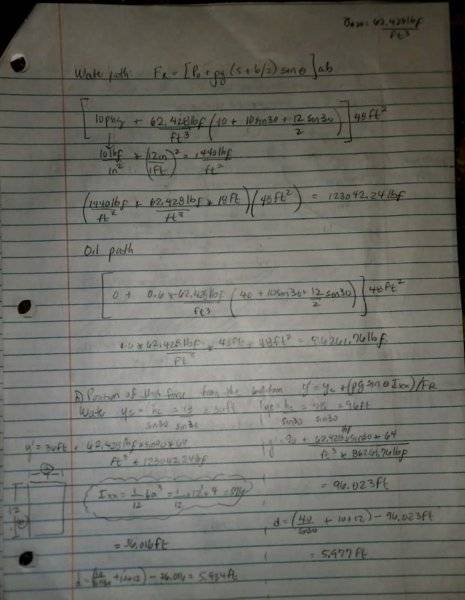# Fluid Mechanics: resultant force of oil and water on a door

## Homework Statement## Homework Equations## The Attempt at a Solution

[/B]My questions:

1. By resultant force, does that mean the force exerted on the side of the water + force exerted on side of the oil? Because I'd think that the force on the side of the water would be different than the force exerted on the door on the side of the oil.

2. When I find the force exerted by the oil, I multiple the SG of the oil by the density of water to get the specific weight of the oil, but in chegg solutions I've been seeing people use 31.75lb/ft^3 and saying that's the specific weight of AIR. Can I use the density of water?

3. When finding the force exerted by the water on the door, I should be considering the 10psig of the air, right?

4. When finding Ixx, I should consider the way the door will open, right? How do I know which way it'll open? I was taking b=12 for Fr so I carried that convention when doing Ixx and used 1/12ba^3 instead of 1/12b^3a which was what I was seeing in the chegg solutions.

Any help is greatly appreciated.

#### Attachments

Last edited by a moderator:

jambaugh
Gold Member
[...]
My questions:

1. By resultant force, does that mean the force exerted on the side of the water + force exerted on side of the oil? Because I'd think that the force on the side of the water would be different than the force exerted on the door on the side of the oil.
Yes. (as vectors or at least magnitude and indicated direction.) and yes, the two forces will be different in general. The door is locked in place and presumably if unlocked would open one way or the other depending on the net resultant force.

2. When I find the force exerted by the oil, I multiple the SG of the oil by the density of water to get the specific weight of the oil, but in chegg solutions I've been seeing people use 31.75lb/ft^3 and saying that's the specific weight of AIR. Can I use the density of water?
You should work out the force from each side independently and then (vectorally as in pay attention to direction) add them. The presence of the door isolates the two sides so nothing about the water side affects the oil side et vice versa.
3. When finding the force exerted by the water on the door, I should be considering the 10psig of the air, right?
Right. As also with the oil you have to consider atmospheric pressure. The only question, and an ambiguity I see in the problem is whether the 10psi is relative to vacuum or relative to atmospheric pressure. i.e. is the pressure above the water Atmospheric pressure plus 10psi or just 10psi. I would assume the simpler case (relative to atmospheric) so that you don't need to input the additional information of what 1 atm is defined to be in psi.
4. When finding Ixx, I should consider the way the door will open, right? How do I know which way it'll open? I was taking b=12 for Fr so I carried that convention when doing Ixx and used 1/12ba^3 instead of 1/12b^3a which was what I was seeing in the chegg solutions.
Any help is greatly appreciated.

I don't think how it will open will matter. The positioning of the force is at the center of force so that the force on the door plus torque around any pivot point will be the same as if all the force were applied at a single point. To find this you'll need the first moment of the force by integrating the differential force due to pressure times the position at which that pressure is being applied. Find the total first moment by adding (including sign of the normal force so really subtracting) the contributions from both water side and oil side. Then divide that moment by the total net force to find the average point at which this total force is being applied.

Chestermiller
Mentor
The only question, and an ambiguity I see in the problem is whether the 10psi is relative to vacuum or relative to atmospheric pressure. i.e. is the pressure above the water Atmospheric pressure plus 10psi or just 10psi. I would assume the simpler case (relative to atmospheric) so that you don't need to input the additional information of what 1 atm is defined to be in psi.
The diagram says 10 psig (i.e., gauge pressure)